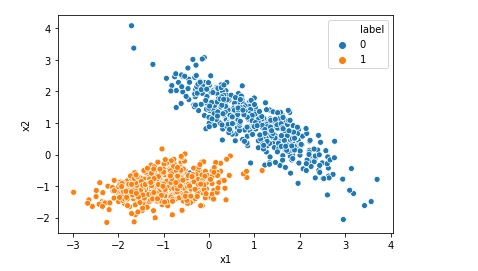# int object is not iterable Error

If your code is like below count = 100 for number in count: This will give you error like.. int object is not iterable. In Python, the thing you pass to a for statement needs to be some kind of iterable object. The variable count here is a number which is not iterable. You should … Continue reading int object is not iterable Error# Visualize or Print Random Forest Algorithm Model

As a machine learning engineer you may have created the Random Forest Algorithm Model, but have you ever tried to visualize it. If not , this is the post for you. In this post we will learn how to Visualize or Print Random Forest Algorithm Model in Jupyter notebook. https://www.youtube.com/watch?v=6uPavHNuatA&t=1s Top 10 Free Books for … Continue reading Visualize or Print Random Forest Algorithm Model# Random Forest Classification in Python in 10 Lines

Random Forest algorithm is like an ensemble algorithm made of Decision Trees, which comprises more than one decision tree to create a model. It creates more than one tree like conditional control statements to create its model hence it is named as Random Forest. Random Forest machine learning algorithm can be used to solve both … Continue reading Random Forest Classification in Python in 10 Lines# Random Forest Regression in Python in 10 Lines

Random Forest algorithm is like Decision Tree, which comprises more than one decision tree to create a model. Random Forest algorithm is an ensemble method. It creates more than one tree like conditional control statements to create its model hence it is named as Random Forest. Random Forest machine learning algorithm can be used to … Continue reading Random Forest Regression in Python in 10 Lines# Visualize or Print Decision Tree Algorithm Model

As a machine learning engineer you may have created the Decision Tree Model, but have you ever tried to visualize it. If not , this is the post for you. In this post we will learn how to Visualize or Print Decision Tree Model in Jupyter notebook. Let us import the required library: from IPython.display … Continue reading Visualize or Print Decision Tree Algorithm Model# Decision Tree Classification in Python in 10 lines

Decision tree machine learning algorithm can be used to solve not only regression but also classification problems. This algorithm creates a tree like conditional control statements to create its model hence it is named as decision tree. In this post we will be implementing a simple decision tree classification model using python and sklearn. First … Continue reading Decision Tree Classification in Python in 10 lines# Print ROC AUC Receiver Operating Characteristic Area Under Curve

The receiver operating characteristic area under curve is a way to measure the performance of a classification model, may be created using algorithms like Logistic Regression. ROC-AUC is basically a graph where we plot true positive rate on y-axis and false positive rate on x-axis. If a model is good the AUC will be close to 1. Area … Continue reading Print ROC AUC Receiver Operating Characteristic Area Under Curve# Scaling Data Range using Min Max Scaler

Suppose you have a dataset that has float values and all values in the range 0 to 1. You want to change all values to integer with a range between 10 to 20. In this post we will learn how to do this using MinMaxScaler Data before scaling Now let us scale the data as … Continue reading Scaling Data Range using Min Max Scaler# Creating Synthetic Data for Logistic Regression

Many a times we want to implement Logistic Regression on certain data but we do not find that kind of data online. In that case we can generate a synthetic data for our problem. In this post we will see how to generate a typical synthetic data for a simple Logistic Regression. Import the required … Continue reading Creating Synthetic Data for Logistic Regression